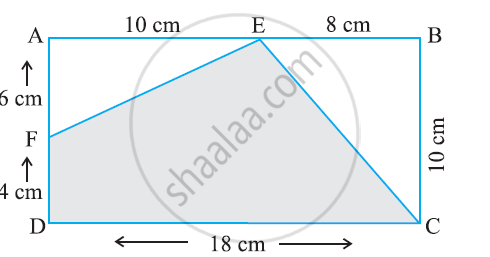Share

# In the Following Figure, Find the Area of the Shaded Portions -1 - CBSE Class 7 - Mathematics

ConceptConcept of Application of Conversion

#### Question

In the following figure, find the area of the shaded portions#### Solution

Area of EFDC = ar (ABCD) − ar (BCE) − ar (AFE)

= (18 × 10) − 1/2 (10 × 8) − 1/2 (6 × 10)

= 180 − 40 − 30 = 110 cm2

Is there an error in this question or solution?

#### APPEARS IN

NCERT Solution for Mathematics for Class 7 (2018 to Current)
Chapter 11: Perimeter and Area
Ex. 11.40 | Q: 10.1 | Page no. 227
Solution In the Following Figure, Find the Area of the Shaded Portions -1 Concept: Concept of Application of Conversion.
S